Physica A: Statistical Mechanics and its Applications
Volume 385, Issue 2, 15 November 2007, Pages 558-572

Thermodynamic variables of microquasars inferred from tachyonic spectral maps

aDepartment of Physics, Hiroshima University, 1-3-1 Kagami-yama, Higashi-Hiroshima 739-8526, Japan

revised 4 June 2007.
Available online 13 June 2007.

### Abstract

Tachyonic spectral densities of ultra-relativistic electron populations are fitted to the γ-ray spectra of two microquasars, LS 5039 and LSI +61°303. The superluminal spectral maps are obtained from BATSE, COMPTEL, EGRET, HESS, and MAGIC data sets. The spectral averaging is done with exponentially cut power-law densities. Estimates of the electron distributions generating the tachyon flux are obtained from the spectral fits, such as power-law indices, electron temperature and source counts. The internal energy and heat capacities of the source populations are calculated. An extensive entropy functional is defined for Boltzmann power-law densities and its stability is checked. The high-temperature limit of the thermodynamic variables is determined by the power-law index of the electron plasma, which enters in the scaling exponents as well as the amplitudes.

Keywords: Superluminal radiation; Tachyonic γ-rays; Boltzmann power-law ensembles; Spectral averaging; γ-Ray binaries

PACS classification codes: 05.70.Ce; 05.20.Gg; 52.25.Kn; 95.30.Tg

### 1. Introduction

We study the tachyonic spectral maps of two microquasars, LS 5039, cf. Refs.  and , and LSI +61°303, cf. Refs.  and , and reconstruct the electron distributions emitting the superluminal radiation. The spectral maps of these γ-ray binaries suggest cascade spectra which are generated by electronic power-law densities exponentially cut with the Boltzmann factor. Classical statistics applies, as the high-temperature regime is invoked at γ-ray energies. We average the tachyonic spectral densities with electronic power-law distributions, and infer the power-law index, the electron count, and the temperature from the spectral fits. Based on these parameters, we calculate the internal energy and the specific heat of the ultra-relativistic electron plasma of the microquasars. To this end, we set up the thermodynamic formalism for Boltzmann power-law ensembles. Starting with the grand partition function, we find an entropy functional for exponentially cut power-law densities that is extensive and stable. We derive the Helmholtz and Gibbs free energies as well as the low- and high-temperature expansions of the caloric equation of state and the heat capacities, in particular their high-temperature scaling depending on the electronic power-law index.

In Section 2, we briefly sketch the transversal and longitudinal tachyonic spectral densities and their averaging with Boltzmann power laws, which results in cascade spectra. We perform the spectral fits to the γ-ray wideband of the microquasars, and extract the parameters of the electronic source populations, such as power-law index, temperature and source number. In Section 3, we develop the thermodynamic formalism for exponentially cut power-law densities and derive the low- and high-temperature expansions of the thermodynamic variables, focusing on entropy, equations of state, and the specific heats. In Section 4, we investigate ultra-relativistic Boltzmann ensembles, power-law densities with Lorentz factors exceeding a high-energy threshold, and we calculate the internal energy and heat capacities of the electron gas in the two microquasars. In Section 5, we present our conclusions. In Appendix A, we list the asymptotic expansions of the partition function, entropy, and the free energies at integer electronic spectral index, which requires special treatment due to logarithmic temperature dependence in the high-temperature regime.

### 2. Tachyonic γ-ray spectra of microquasars

The tachyonic radiation densities of uniformly moving electrons were derived in Ref. ,

where ΔT=1 refers to the transversal superluminal radiation, and ΔL=0 holds for the longitudinal component. We use the classical limit of the radiation densities, neglecting terms depending on the electron–tachyon mass ratio, as the spectral fits are done in the ultra-relativistic high-temperature regime ; γ denotes the electronic Lorentz factor, αq the tachyonic fine structure constant, and mt the tachyon mass. The spectral cutoff occurs at. Only frequencies in the range 0<ω<ωmax(γ) can be radiated by a uniformly moving charge; the tachyonic spectral densities pT,L(ω) are cut off at the break frequency ωmax. We use the Heaviside–Lorentz system, so that αq=q2/(4π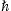c)≈1.0×10-13. The tachyon mass is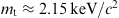, and the tachyon–electron mass ratio mt/m≈1/238. These estimates are obtained from hydrogenic Lamb shifts .

The radiation densities (2.1) refer to a single charge with Lorentz factor γ. We average them with ultra-relativistic electron distributions, power-law densities exponentially cut with the Boltzmann factor:

The electronic Lorentz factors range in an interval γ1γ<∞. The normalizationis determined by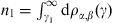, where n1 is the electron count and γ1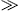1 the lower edge of Lorentz factors in the source population. The exponential cutoff is related to the electron temperature by β=mc2/(kT), and α is the electronic power-law index. When discussing the thermodynamics of Boltzmann power-law ensembles, we will redefine the power-law index as δ=α+2, so that a thermal Maxwell–Boltzmann distribution corresponds to δ=0 and γ1=1. In spectral averages it is customary to define the electron index by α as done in Eq. (2.2). The average is carried out as 

and the spectral fit is based on the E2-rescaled flux densities:

where d is the distance to the source. The spectral maps of the microquasars in Fig. 1, Fig. 2 and Fig. 3 are fitted with the unpolarized flux density dNT+L=dNT+dNL of electron populations ρi=1,2 specified in Table 1. Each electron density generates a cascade ρi, and the wideband fit is obtained by adding two cascade spectra, labeled ρ1 and ρ2 in the figures.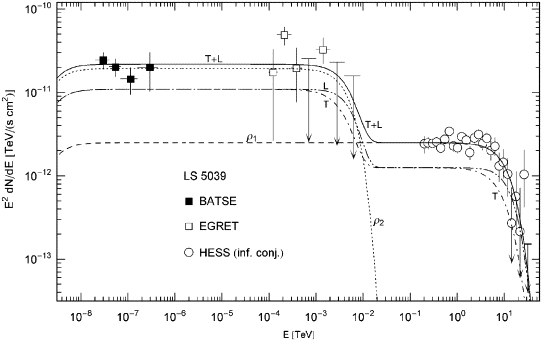Fig. 1. γ-Ray wideband of the microquasar LS 5039. BATSE data points from Ref. , EGRET points from Refs.  and , and HESS points from Ref. . BATSE and EGRET data refer to the associated EGRET source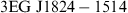, HESS points to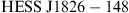at the inferior conjunction as defined in Ref. . The solid line T+L depicts the unpolarized differential tachyon flux dNT+L/dE, obtained by adding the flux densities ρ1,2 of two electron populations and rescaled with E2 for better visibility of the spectral curvature, cf. (2.4). The transversal (T, dot-dashed) and longitudinal (L, double-dot-dashed) flux densities dNT,L/dE add up to the total flux T+L. The exponential decay of the cascades ρ1,2 sets in at about Ecut≈(mt/m)kT, implying cutoffs at 5 TeV for the ρ1 cascade and at 2 GeV for ρ2, which terminate the spectral plateaus. The unpolarized flux T+L is the actual spectral fit, the parameters of the electron densities are recorded in Table 1.Fig. 2. Close-up of the HESS spectrum of LS 5039 in Fig. 1. The TeV spectral map coincides with the ρ1 cascade, since the ρ2 flux is exponentially cut at 2 GeV. T and L stand for the transversal and longitudinal flux components, and T+L labels the unpolarized flux. The HESS points define a spectral plateau in the high GeV range typical for cascade spectra, followed by exponential decay. The spectral curvature is generated by the Boltzmann factor in the electron densities. If rescaled, this spectral map is quite similar to the cascade spectra of the Markarian galaxies Mkn 501 and Mkn 421 in Figs. 5 and 6 of Ref. .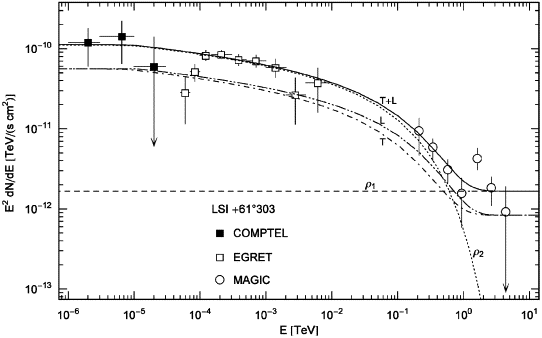Fig. 3. Spectral map of the microquasar LSI +61°303, associated with the EGRET source 3EG J0241+6103. COMPTEL points from Ref. , EGRET data from Refs.  and , and MAGIC points from Ref. . The plots are labeled as in Fig. 1. The χ2-fit is done with the total unpolarized tachyon flux T+L and subsequently split into transversal (dot-dashed) and longitudinal (double-dot-dashed) components. A spectral break at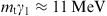is visible as edge in the spectral map; exponential decay sets in at the cutoff frequency. In the extended crossover regime between the spectral break and the spectral cutoff, the decay is gradual but not power law, with electron index α=1. (A power-law crossover,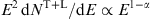, occurs for electron indices α>1, cf. Ref. ).

Table 1.

Electronic source densities ρ1,2 generating the γ-ray broadband of the microquasars LS 5039 and LSI +61°303

α β γ1ne kT (TeV)
LS 5039 @2.5 kpc
ρ1 −2 4.39×10−10 1 2.7×10−4 9.7×1046 1160
ρ2 −2 1.075×10−6 1 2.1×10−3 7.6×1047 0.475
LSI +61°303 @2 kpc
ρ1 −2 1 1.8×10−4 4.1×1046
ρ2 1 3.77×10−9 5.0×103 1.2×10−2 2.8×1048 136

Each ρi stands for a Boltzmann power-law density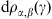defined by parameters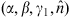, cf. (2.2). α is the electronic power-law index, β=mc2/(kT) the cutoff parameter in the Boltzmann factor, and γ1 the lower edge of Lorentz factors in the electronic source population ρi. The amplitude of the tachyon flux generated by ρi is determined by, from which the electron count ne is inferred at the indicated distance, cf. (2.5). (The subscript 1 in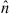and ne has been dropped). kT is the electron temperature. In the thermodynamic variables, we identify δ=α+2 and put N=ne, cf. Sections 3 and 4. The cascades labeled ρi in Fig. 1, Fig. 2 and Fig. 3 are obtained by averaging the tachyonic radiation densities (2.1) with the electron distributions ρi, cf. (2.3) and (2.4). The parameters α, β, γ1, andare extracted from the least-squares fit T+L in the figures. As for the thermal high-energy population ρ1 of LSI +61°303, it is not yet possible to determine the spectral cutoff and the electron temperature from the presently available TeV data.

As for the electron count n1, we use a rescaled parameterfor the fit:

which is independent of the distance estimate. Here,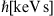implies the tachyon mass in keV units, that is, we put mt≈2.15 in the spectral density (2.1). At γ-ray energies, only a tiny αq/αe-fraction (the ratio of tachyonic and electric fine structure constants) of the tachyon flux is absorbed by the detector, which requires a rescaling of the electron count n1, so that the actual number of radiating electrons is. We thus find the electron count as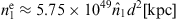, where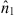defines the tachyonic flux amplitude extracted from the spectral fit  and . As for electron temperature and cutoff parameter in the Boltzmann factor, we note kT[TeV]≈5.11×10-7/β. The electronic source count for the Crab pulsar at d≈2 kpc is 2.6×1049, cf. Ref. , as compared to 8.6×1047 for LS 5039 and 2.8×1048 for LSI +61°303; these estimates are obtained by adding the source counts of the high- and low-energy populations ρ1,2 in Table 1. A similar source number of 3.2×1047 was derived in Ref.  for the binary pulsarat 1.5 kpc, which suggests a neutron star as compact companion in both microquasars.

In Fig. 1 and Fig. 2, we depict the spectral map of the microquasar LS 5039, located at a distance of 2.5 kpc, a compact object orbiting a massive O-star , ,  and . Mass estimates and orbital parameters are given in Refs.  and ; we do not list them here, as they are not required to infer the thermodynamic parameters of the electron plasma. LS 5039 is associated with the unidentified EGRET sourceand the TeV source, cf. Refs.  and . The tachyonic spectral maps are further explained in the figure captions. The GeV spectrum in Fig. 1 can be compared to the spectral map in Fig. 2 of Ref. , based on inverse Compton scattering.

Fig. 3 shows the tachyonic spectral map of the microquasar LSI +61°303, at d≈2 kpc, associated with the unidentified EGRET source 3EG J0241+6103, cf. Refs. , , , ,  and . Estimates of the orbital parameters of this binary system, a massive Be star with a compact companion, are given in Refs.  and . Inverse-Compton fits of the EGRET spectrum can be found in Figs. 2–4 of Ref. . The thermodynamic variables of the electron populations are discussed in Section 3, and estimates of the internal energy and the specific heats are derived in Section 4. The spectral fits in Fig. 1, Fig. 2 and Fig. 3 are performed with the unpolarized tachyon flux. At γ-ray energies, the speed of tachyons is close to the speed of light, the basic difference to electromagnetic radiation being the longitudinal flux component. The polarization of tachyons can be determined from transversal and longitudinal ionization cross-sections  and .

### 3. Caloric equation of state and heat capacities of electronic power-law distributions

The exponentially cut power-law distribution (2.2) is obtained from the momentum-space density dρH-δe-H/(kT)d3p, where H= is the free Hamiltonian, and the electronic Lorentz factors range in the interval γ1γ<∞. The power-law exponent δ is related to the electron index in Eq. (2.2) by δ=α+2. The thermodynamic formalism employed is standard, and no derivations are given, although we will consider stability conditions such as the positivity of the specific heat and compressibility. We will discuss the low- and high-temperature expansions of the caloric equation of state and the isochoric heat capacity, in particular their qualitative dependence on the power-law index δ. The typical range of δ in spectral averages is narrow, very rarely outside the interval 0δ4, cf. Refs. , , , , , , ,  and . The relativistic thermal Maxwell–Boltzmann distribution in 3D is recovered with δ=0 and γ1=1, cf. Ref. . Here, we will study power-law ensembles with arbitrary real index δ.

with density

where H/m=γ and β=m/(kT). The thermal wavelength reads, andis required for classical statistics to apply. We parametrize the momentum integration in Eq. (3.1) with the Lorentz factor,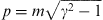, and perform the angular integration, so that. In the ultra-relativistic limit, p, the factor γδ in density (3.2) can be generated by analytic continuation in the space dimension. The fact that the exponent δ varies only in a narrow range also suggests treating it like a dimension rather than an intensive variable when setting up the thermodynamic formalism for power-law ensembles. We find

where

The momentum integration (3.3) is over R3, as we at first consider the full range of Lorentz factors, 1γ<∞. In Section 4, we will discuss a truncated electron density, restricting the integration range in Eq. (3.3) to HH1=mγ1, γ1>1, which amounts to replacing the lower integration boundary in Eq. (3.4) by γ1; in particular, we will study ultra-relativistic ensembles with high-energy threshold γ11. In this section, we assume γ1=1.

The summation in Eq. (3.1) gives

and we find the internal energy and the particle number as

Here, m3V is dimensionless, so that mmc/when restoring units, and β=mc2/(kT). On eliminating the fugacity e-α, we obtain the caloric equation of state:

and the entropy is defined as

By making use of N=log Z, we find

The homogeneity relation, S/N=S(δ,β,V/N,1), is satisfied, and we may substitute β(U/N) by solving (3.8).

We note the Helmholtz free energy UTS,

and the thermal equation of state, P=-∂F/∂V or PV=mN/β. The isochoric specific heat reads

This is the energy required to shift the exponential cutoff of the power-law slope, that is, to vary the electron temperature without changing volume and power-law index. The isothermal compressibility is

Thermodynamic stability, d2S0 at dS=0 (with δ kept fixed) requires CV0 and κT0, the latter is obviously satisfied. As for the first, we note K(δ,β)/∂β=-K(δ-1,β) and the Schwarz inequality K2(δ-1,β)<K(δ,β)K(δ-2,β), which implies CV>0. As for energy and number fluctuations, we note the variances

which result in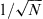decay of the fluctuations ΔU/U and ΔN/N. The isobaric expansivity is

where, and the isobaric heat capacity CP is calculated by means of the identity

and are calculated via the identities 

where γC denotes the heat capacity ratio CP/CV. The positivity conditions CV>0 and κT>0 thus imply CP>CV, γC>1, and κT>κS>0; the adiabatic expansivity αS is negative.

The low-temperature expansion of the equation of state (3.8) reads

As for the heat capacities at constant volume/pressure, we use (3.13) to find

The leading order is just the non-relativistic result; at low temperature, the power-law index enters only into the relativistic corrections. Here and in the following, the asymptotic expansions are obtained by term-by-term differentiation of the log K series in Appendix A.

The high-temperature expansions of the internal energy and the isochoric specific heat differ qualitatively in different δ ranges. We define the coefficients

and find, for δ<1,

In the range 1<δ<3, the next-to-leading order is modified as

In the interval 3<δ<4, we obtain

and for 4<δ<5

Finally, for δ>5

The leading-order coefficients are all positive, which is easy to see except for CV in Eq. (3.27), where positivity follows from the Schwarz inequality, cf. after (3.14). Regarding units, we note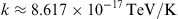and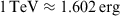.

In the case of integer δ, there appear singularities in the expansion coefficients, which cancel if ε expanded, cf. Appendix A and Ref. . We list the equation of state and the specific heat for integer power-law index 0δ5. Outside this range, the indicated orders of expansions (3.23) and (3.27) are singularity free and can be used even at integer δ. As for the caloric equation, we find

where γE≈0.5772. The internal energy admits a finite high-temperature limit for power-law indices δ>4. The enthalpy is U+mN/β. The high-temperature expansion of the corresponding isochoric heat capacity reads

The case δ=0 is the high-temperature limit of a Maxwell–Boltzmann distribution . The specific heat is safely positive, but approaches zero in the high-temperature limit for power-law indices δ3.

### 4. Ultra-relativistic Boltzmann power laws

We proceed as in Section 3, but restrict the momentum integration in the partition function (3.1) to ultra-relativistic Lorentz factors, so thatwith γ ranging above a high-energy edge γ11. In the partition function (3.1), we substitute the truncated density

where H1=mγ1 is the threshold energy. The step function restricts the d3pd3q integration in Eq. (3.1) to H(p,q)H1:

with density ρ in Eq. (3.2), so that

Eqs. (3.5), (3.6), (3.7), (3.8), (3.9), (3.10), (3.11), (3.12), (3.13), (3.14), (3.15), (3.16), (3.17), (3.18) and (3.19) remain valid with K(δ,β) replaced by K(δ,β,γ1); internal energy and heat capacity read

Schwarz's inequality as stated after (3.14) also applies to K(δ,β,γ1), so that the maximum condition d2S0 at fixed δ and γ1 is met. The low-temperature expansion (3.20) of the internal energy is replaced by 

where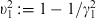. The asymptotic parameter is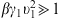, so that this expansion remains valid for small β and large βγ1. The low-temperature limit of the heat capacity (4.5) reads

If γ1≈1, these expansions break down; the low-temperature expansion of K(δ,β,γ1) in the case of small but non-vanishing υ1 is discussed in Ref. . At γ1=1, the low-temperature expansions (3.20) and (3.21) apply. In this section, we consider ultra-relativistic power-law ensembles, with Lorentz factors exceeding a high-energy threshold γ11.

The high-temperature expansion of the internal energy and the specific heat as stated in Eqs. (3.23), (3.24), (3.25), (3.26) and (3.27) can also be used for γ1>1, if we replace the coefficients ck(δ) in Eq. (3.22) by

We note ck(δ,1)=ck(δ). In the ultra-relativistic limit, γ11, the hypergeometric function in ck(δ,γ1) can be dropped, cf. (A.21). However, care must be taken if the power-law exponent δ is integer, as singularities emerge in the coefficients of the hypergeometric series, cf. (A.19), (A.20), (A.21), (A.22) and (A.23). For integers 0δ5 and ultra-relativistic γ11, the high-temperature expansions of the internal energy read

The corresponding high-temperature expansions of the ultra-relativistic heat capacity at integer power-law index 0δ5 are

The expansions in Eqs. (4.9) and (4.10) are ultra-relativistic, terms of orderare systematically dropped, cf. (A.22). The high-temperature expansions valid for γ1=1 are stated in Eqs. (3.28) and (3.29). In Table 2, we list the internal energy and heat capacities of the electron populations in the microquasars LS 5039 and LSI +61°303. The input parameters are inferred from the spectral fits in Fig. 1, Fig. 2 and Fig. 3, as explained in the table captions.

Table 2.

Internal energy and heat capacities of the electronic source densities ρ1,2 in the microquasars

U/(nemc2) U (erg) CV (erg/K) CP/CV
LS 5039
ρ1 6.83×109 5.4×1050 4.0×1031 1.33
ρ2 2.79×106 1.7×1048 3.1×1032 1.33
LSI +61°303
ρ1 1.7×1031 1.33
ρ2 2.57×107 5.9×1049 3.4×1031 12.4

U/(nemc2) denotes the internal energy normalized with the rest mass of the respective electron population ρi. The internal energy U (erg) of the thermal densities ρ1,2 in LS 5039 and ρ1 in LSI +61°303 is calculated via the high-temperature expansion U(δ=0) in Eq. (3.28). The series U(δ=3,γ1) in Eq. (4.9) is used for the ultra-relativistic power-law density ρ2 of LSI +61°303. The input parameters δ=α+2, β, γ1, and N=ne are listed in Table 1. The isochoric heat capacity is calculated by means of the series expansions CV(δ=0) in Eq. (3.29) and CV(δ=3,γ1) in Eq. (4.10). The adiabatic index CP/CV is obtained from identity (3.17). The internal energy of the thermal population ρ1 in LSI +61°303 remains undetermined, as the cutoff parameter β of this distribution could not be extracted from the spectral fit in Fig. 3. Regarding the ultra-relativistic power-law density ρ2 of LSI +61°303, we note βγ1≈1.9×10-5, cf. Table 1, so that the quoted high-temperature expansions of internal energy and specific heat are applicable, obtained from the ultra-relativistic expansion K(δ=3,β,γ1) listed in Eq. (A.22).

### 5. Conclusion

We have investigated superluminal radiation from ultra-relativistic electron populations in γ-ray binaries and explained how to obtain the thermodynamic variables of the source densities. We demonstrated that the γ-ray spectra of the microquasars can be fitted with tachyonic cascade spectra. The spectral curvature is intrinsic and reproduced by the tachyonic spectral densities (2.1) averaged with thermal and power-law electron distributions. In Table 2, we have given estimates of the internal energy and the heat capacities of the electron plasmas in the microquasars LS 5039 and LSI +61°303. More generally, we have shown that Boltzmann power-law densities admit a stable and extensive entropy function (3.11). On that basis, we have derived the caloric equation of state and studied the temperature scaling of the internal energy and the heat capacities in the high-temperature regime, in particular the dependence of the scaling exponents on the electronic power-law index.

The tachyonic radiation densities (2.1) are generated by electrons in uniform motion. In Ref. , we studied superluminal radiation from ultra-relativistic electrons orbiting in strong magnetic fields and derived the pitch-angle scaling of the radiation densities. In the zero-magnetic-field limit, the tachyonic synchrotron densities converge to the spectral densities for uniform motion . Orbital curvature induces modulations in the spectral slopes of densities (2.1), but these ripples are attenuated when performing a pitch-angle average, cf. Figs. 1–3 in Ref. . Thus we can use uniform radiation densities in the spectral fits even in the presence of magnetic fields, for instance, if the compact companion is a magnetized neutron star  and . The orbital parameters of the binary system do not directly enter into the spectral fit either. If one considers microquasars as scaled-down Galactic counterparts of active galactic nuclei  and , one would rather expect the spectra to be determined by the global thermodynamic parameters of the electron populations in these objects, which are in turn inferred from the spectral maps, cf. Table 1.

We have focused on γ-ray spectra, which can be fitted with ultra-relativistic electron populations in the high-temperature regime. Otherwise we have to consider the quantized tachyonic spectral densities  instead of the classical limit (2.1). In the low-temperature regime, we also have to replace the Boltzmann power-law density in the spectral average (2.3) with its quantized fermionic counterpart. As for tachyonic X-ray spectra obtained from diffraction gratings, the tachyon mass of 2 keV has to be included in the dispersion relation,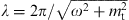, when parametrizing the glancing angle in the Bragg condition with energy, which affects the shape of the spectral maps in the X-ray bands. This will be discussed elsewhere.

### Acknowledgements

The author acknowledges the support of the Japan Society for the Promotion of Science. The hospitality and stimulating atmosphere of the Centre for Nonlinear Dynamics, Bharathidasan University, Trichy, and the Institute of Mathematical Sciences, Chennai, are likewise gratefully acknowledged.

### References

 M.V. McSwain et al., Astrophys. J. 600 (2004), p. 927.

 J. Casares et al., Mon. Not. R. Astron. Soc. 364 (2005), p. 899.

 J. Casares et al., Mon. Not. R. Astron. Soc. 360 (2005), p. 1105.

 E.D. Grundstrom et al., Astrophys. J. 656 (2007), p. 437.

 R. Tomaschitz, Physica A 320 (2003), p. 329.

 R. Tomaschitz, Eur. Phys. J. C 49 (2007), p. 815.

 R. Tomaschitz, Ann. Phys. 322 (2007), p. 677.

 B.A. Harmon et al., Astrophys. J. Suppl. 154 (2004), p. 585.

 V. Bosch-Ramon and J.M. Paredes, Chin. J. Astron. Astrophys. 5 (Suppl.) (2005), p. 133.

 V. Bosch-Ramon and J.M. Paredes, Astron. Astrophys. 417 (2004), p. 1075.

 F. Aharonian et al., Astron. Astrophys. 460 (2006), p. 743.

 R. van Dijk et al., Astron. Astrophys. 315 (1996), p. 485.

 D.A. Kniffen et al., Astrophys. J. 486 (1997), p. 126.

 V. Bosch-Ramon and J.M. Paredes, Astron. Astrophys. 425 (2004), p. 1069.

 J. Albert et al., Science 312 (2006), p. 1771.

 R. Tomaschitz, Astropart. Phys. 27 (2007), p. 92.

 R. Tomaschitz, Phys. Lett. A 366 (2007), p. 289.

 J.M. Paredes, V. Bosch-Ramon and G.E. Romero, Astron. Astrophys. 451 (2006), p. 259.

 M. Chernyakova, A. Neronov and R. Walter, Mon. Not. R. Astron. Soc. 372 (2006), p. 1585.

 L. Sidoli et al., Astron. Astrophys. 459 (2006), p. 901.

 R. Tomaschitz, Eur. Phys. J. D 32 (2005), p. 241.

 R. Tomaschitz, J. Phys. A 38 (2005), p. 2201.

 J.M. Fierro et al., Astrophys. J. 494 (1998), p. 734.

 S.P. Reynolds and J.W. Keohane, Astrophys. J. 525 (1999), p. 368.

 I. de Pater et al., Icarus 163 (2003), p. 434.

 K. Tsuchiya et al., Astrophys. J. 606 (2004), p. L115.

 C. Itoh et al., Astron. Astrophys. 462 (2007), p. 67.

 R. Tomaschitz, Physica A 335 (2004), p. 577.

 R. Tomaschitz, Astropart. Phys. 23 (2005), p. 117.

 F. Aharonian et al., Astron. Astrophys. 430 (2005), p. 865.

 R. Enomoto et al., Astrophys. J. 652 (2006), p. 1268.

 S. Chandrasekhar, An Introduction to the Study of Stellar Structure, Dover, New York (1967).

 H.B. Callen, Thermodynamics and an Introduction to Thermostatistics, Wiley, New York (1987).

 R. Tomaschitz, Eur. Phys. J. C 45 (2006), p. 493.

### Appendix A. High-temperature asymptotics of Gibbs free energy and entropy

The low- and high-temperature expansions of the partition function

amount to deriving the β→∞ and β→0 asymptotics of the integral

The first few orders of the low-temperature expansion read 

The high-temperature expansion is composed of two series:

where

The general term of the series indicated in Eq. (A.5) is (-)k(1/2)kΓ(3-δ-2k)β2k/k!, where (1/2)k denotes the falling factorial, cf. Ref. . The second series in Eq. (A.4) reads

with coefficients ck(δ) defined in Eq. (3.22). To get an overview, we summarize the leading orders of expansion (A.4), with power-law index δ ranging in the indicated intervals:

At integer δ, pole singularities emerge in the coefficients of series (A.5) and (A.6), which cancel in Eq. (A.4) if ε expanded :

γE is Euler's constant. At integer δ<0 and δ>5, expansions K(δ<1,β) and K(δ>5,β) in Eq. (A.7) can be used, as the indicated orders are singularity free. This settles the high-temperature expansion of the partition function (A.1) for arbitrary power-law index δ.

The high-temperature expansion of the chemical potential (3.9), the Helmholtz free energy (3.12) and the Gibbs potential F+PV,

are obtained from the logK(δ,β) series, which reads in the respective δ-range as

Internal energy and specific heat are found by term-by-term differentiation of the log K series. For integers 0δ5, singularities occur in the series coefficients in Eq. (A.10). In this case, we use (A.8) to find

The high-temperature expansion of the entropy function (3.11) is obtained by substituting the asymptotic series of the caloric equation of state and the chemical potential into

Alternatively, we may use the log K series in Eqs. (A.10) and (A.11) and term-by-term differentiation to find the β→0 asymptotics of S, starting with

and S0 approaches a finite limit,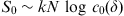, for δ>3, cf. (A.10). At δ=3, S0 has a double-logarithmic divergence in the high-temperature limit, cf. (A.11).

We finally discuss the low- and high-temperature asymptotics of the ultra-relativistic partition function, cf. (4.1):

We find log Z as in Eq. (A.1), with K(δ,β) replaced by

so that

Here, N is to be identified with the renormalized electron count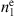as defined after (2.5), and the power-law exponent δ is related to the electron index α defined in Eq. (2.2) by δ=α+2. The low-temperature expansions of the chemical potential (3.9) and the free energies (3.12) and (A.9) are obtained by substituting 

where.

It remains to settle the high-temperature limit, the β→0 asymptotics of K(δ,β,γ1) in Eq. (A.16). As in Eq. (A.4), the expansion is composed of two series :

where seriesis defined in Eq. (A.5) and

with coefficients ck(δ,γ1) defined in Eq. (4.8). The high-temperature expansion of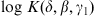reads as stated in Eq. (A.10) for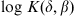, with coefficients ck(δ) replaced by ck(δ,γ1). In the ultra-relativistic limit, we can use the hypergeometric series in Eq. (4.8):

so that the expansion parameter of series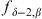in Eq. (A.20) is βγ1. If βγ11, despite of β being small, the low-temperature expansion (A.18) applies.

At integer δ, the coefficients ck(δ,γ1) in Eq. (A.20) become singular owing to poles in the coefficients of the hypergeometric series. They can be dealt with by ε expansion and are canceled by corresponding poles of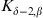in Eq. (A.19), which arise in the Γ-functions in Eq. (A.5). In the ultra-relativistic limit, γ11 (but still βγ1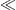1), we find, for integers 0δ5,

There are two expansion parameters in these series, β inand βγ1 in, cf. (A.19). It is possible to perform a systematic high-temperature expansion in β, keeping γ1 fixed and assuming β→0, just by reordering the terms in Eq. (A.22):

Here, we have dropped the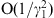estimates indicated in Eq. (A.22). These expansions are valid in the ultra-relativistic regime, γ11, provided that β is sufficiently small to ensure βγ11.

The ultra-relativistic high-temperature asymptotics of entropy is obtained by means of (A.13), with K(δ,β) replaced by K(δ,β,γ1) in the reduced entropy function S0. For power-law indices δ3, the leading order of S0 is independent of the ultra-relativistic threshold γ1, cf. (A.23), so that the high-temperature limit of S0 in Eq. (A.14) remains unchanged. For power-law indices δ>3, the reduced entropy admits a finite limit,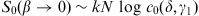, cf. (A.10) and (A.21).Tel.: +81 824 247361; fax: +81 824 240717.